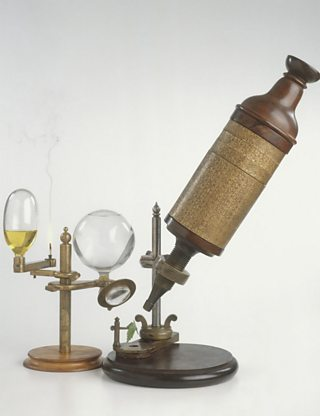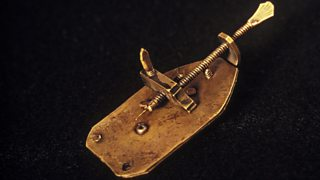# Light microscopes

Greg Foot explains the main differences between light and electron microscopesA replica of Robert Hooke's compound microscope

We need microscopes to study most cells. Microscopes are used to produce magnified images. There are two main types of microscope:

• light microscopes are used to study living cells and for regular use when relatively low and is enough
• electron microscopes provide higher magnifications and higher resolution images but cannot be used to view living cells

## How have light microscopes developed?

Glass was developed by the Romans in the first century. Since then, scientists have been trying to magnify objects. No-one knows who first invented the microscope, but there have been key stages in their development:

1590s: Dutch spectacle maker Janssen experimented with putting lenses in tubes. He made the first compound microscope. None of these microscopes have survived, but they are thought to have magnified from ×3 to ×9.Antonie van Leeuwenhoek's first microscope

1650s: British scientist, Robert Hooke (also famous for his law of elasticity in Physics) observed and drew cells using a .

Late 1600s: Dutch scientist Antonie van Leeuwenhoek constructed a microscope with a single spherical lens. It magnified up to ×275.

The optical quality of lenses increased and the microscopes are similar to the ones we use today. Throughout their development, the magnification of has increased, but very high magnifications are not possible. The maximum magnification with a light microscope is around ×1500.

## Components of light microscopes

The components of a light microscope and their functions

## Calculating the magnification of light microscopes

The compound microscope uses two lenses to magnify the specimen: the eyepiece and an objective lens.

In most microscopes, there is a choice of objectives to use. Magnification can therefore be varied, according to the size of the specimen to be viewed and the level of detail required.

The magnification of a lens is shown by a multiplication sign followed by the amount the lens magnifies. So a lens magnifying ten times would be ×10. The total magnification of a microscope is:

Magnification of the microscope = magnification of eyepiece × magnification of objective

So, if the magnification of an eyepiece is ×10 and the objective is ×4, the magnification of the microscope is:

Magnification of eyepiece × magnification of objective = 10 × 4 = 40

Question

If the magnification of an eyepiece is ×10 and the objective is ×40, what is the magnification of the microscope?

×400. Because 10 × 40 = 400.

## Calculating the magnification of an image

Microscopes use lenses to magnify the image of a specimen so that it appears larger.

The formula to calculate magnification is:

$\text{magnification} = \frac{\text{size of image}}{\text{real size of object}}$

The same formula shown in a magnification triangle:

## Calculating the magnification of a cell

The real size of the cell shown above is 0.05 mm (= 50 μm).

In a book, a photomicrograph of the cell measured 100 mm.

To calculate the magnification:

$\text{magnification} = \frac{\text{100 mm}}{\text{0.05 mm}} = 2000$

It is important to work in the same units when calculating magnification.

Sizes of most cells are given in micrometres, symbol μm.

Calculating the magnification, working in micrometres:

First convert the 100 mm into micrometres:

1 mm = 1000 μm

100 mm = 100 × 1000 μm = 100 000 μm

The magnification of the image calculated in μm:

$\text{magnification} = \frac{\text{100 000 mm}}{\text{50 μm}} = 2000$